#### Differential equations

We now introduce some basic differential equations to model motions. The resulting description is often called a dynamical system. The first step is to describe rigid body velocities in terms of state. Returning to models that involve one or more rigid bodies, the state corresponds to a finite number of parameters. Let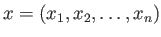(8.18)

denote an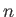-dimensional state vector. If each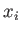corresponds to a position or orientation parameter for a rigid body, then the state vector puts all bodies in their place. Let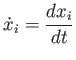(8.19)

represent the time derivative, or velocity, for each parameter.

To obtain the state at any time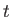, the velocities need to be integrated over time. Following (8.4), the integration of each state variable determines the value at time: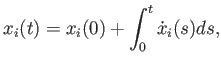(8.20)

in which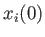is the value ofat time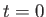.

Two main problems arise with (8.20):

1. The integral almost always must be evaluated numerically.
2. The velocity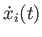must be specified at each time.

Steven M LaValle 2020-11-11